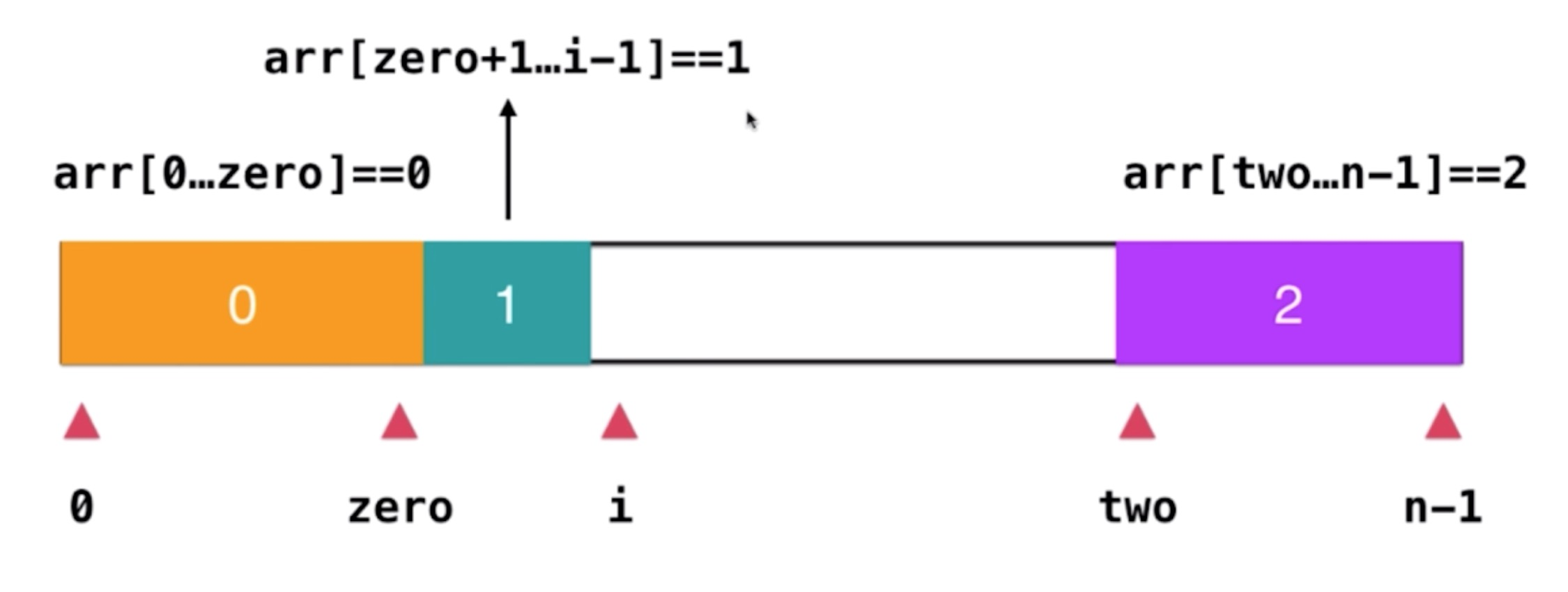Time Flying### 题目

输入: [2,0,2,1,1,0]输出: [0,0,1,1,2,2]

### 解题

输入[2,0,2,1,1,0]输出[0,1,2,1,1,0]预期结果[0,0,1,1,2,2]
/** * @param {number[]} nums * @return {void} Do not return anything, modify nums in-place instead. */var sortColors = function (nums) {  let countObj = {}  for (let i = 0; i < nums.length; i++) {    const key = nums[i]    if (typeof(countObj[${key}]) === 'number') { countObj[${key}] = countObj[${key}] + 1 } else { countObj[${key}] = 1    }  }
const count0 = countObj || 0  const count1 = countObj || 0  const count2 = countObj || 0  for (let i = 0; i < count0 + count1 + count2; i++) {    if (i < count0) {      nums[i] = 0    } else if (i >=count0 && i < count0 + count1) {      nums[i] = 1    } else if (i >= count1 && i < count0 + count1 + count2) {      nums[i] = 2    }  }}/** * @param {number[]} nums * @return {void} Do not return anything, modify nums in-place instead. */var sortColors = function (nums) {  /**   * nums[0, zero] = 0   * nums[two, nums.length - 1] = 2   */  let [zero, two] = [0, nums.length - 1]  for (let i = 0; i <= two; i++) {    if (nums[i] === 0) {      [nums[i], nums[zero]] = [nums[zero], nums[i]]      zero++    }
if (nums[i] === 2) {      [nums[i], nums[two]] = [nums[two], nums[i]]      i--      two--    }  }}

88、215